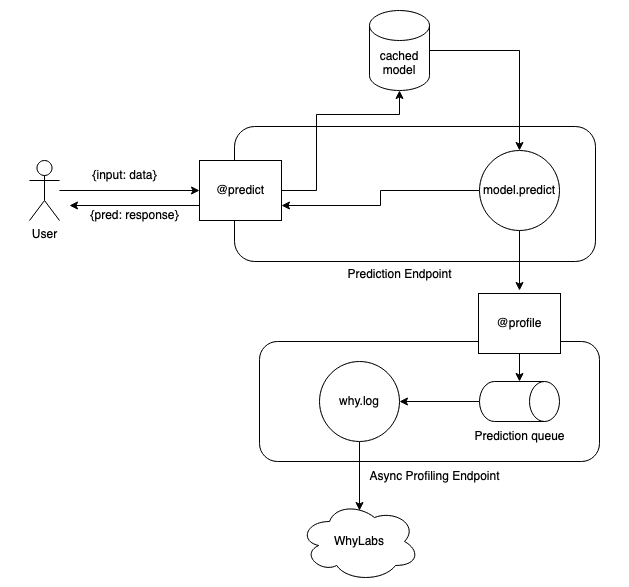# FastAPI

In this section we will learn how to integrate whylogs into a FastAPI server that uploads profiles to WhyLabs.

## Profiling in a prediction endpoint#

The following is an example of a simple prediction endpoint.

import numpy as np
import pandas as pd
from sklearn.neighbors import KNeighborsClassifier
from fastapi import FastAPI
from pydantic import BaseModel
from fastapi.encoders import jsonable_encoder
from fastapi.responses import JSONResponse
CLASS_NAMES = ["setosa", "versicolor", "virginica"]
MODEL_PATH = "s3:///path/to/model.pkl"
app = FastAPI()
class PredictRequest(BaseModel):
sepal_length: float
sepal_width: float
petal_length: float
petal_width: float
def make_prediction(features: pd.DataFrame) -> Tuple:
results = model.predict(features)
probs = model.predict_proba(features)
result = results
output_cls = CLASS_NAMES[result]
output_proba = max(probs)
return (output_cls, output_proba)
@app.post("/predict")
def predict(request: PredictRequest) -> JSONResponse:
data = jsonable_encoder(request)
pandas_df = pd.json_normalize(data)
predictions = make_prediction(features=pandas_df)
pandas_df[["output_class", "output_probability"]] = predictions
return JSONResponse(content=pandas_df.to_dict())

To get the above endpoint integrated with WhyLabs, we will need to:

1. Start a logger, which will keep a profile in memory up until when it's time to merge it
2. Profile the output DataFrame with `whylogs`
3. Close the logger when the app is shutdown
import os
import whylogs as why
os.environ["WHYLABS_DEFAULT_ORG_ID"] = "my_org_id"
os.environ["WHYLABS_DEFAULT_DATASET_ID"] = "my_model_id"
os.environ["WHYLABS_API_KEY"] = "my_key"
@app.on_event("startup")
def start_logger():
global logger = why.logger(mode="rolling", interval=1, when="H", base_name="fastapi_predictions")
logger.append_writer("whylabs")
@app.post("/predict")
def predict(request: PredictRequest) -> JSONResponse:
data = jsonable_encoder(request)
pandas_df = pd.json_normalize(data)
predictions = make_prediction(features=pandas_df)
pandas_df[["output_class", "output_probability"]] = predictions
logger.log(pandas=pandas_df)
return JSONResponse(content=pandas_df.to_dict())
@app.on_event("shutdown")
def close_logger():
logger.close()

⚠️ Best practice is to have these environment variables set on the machine/environment level (such as per the CI/QA machine, a Kubernetes Pod, etc.) to avoid checking those credentials into source control.

### Decoupling whylogs from FastAPI#

In general, whylogs is quite fast with bulk logging, but it does have fixed overhead per log call, so some traffic patterns may not lend themselves well to logging synchronously. If you can't afford the additional latency overhead that whylogs would take in your inference pipeline then you should consider decoupling whylogs.

Instead of directly logging the data on every call, you can send the data to a message queue like SQS to asynchronously log.You can also use our whylogs container to host a dedicated profiling endpoint. You would be creating IO bound rest calls on each inference rather than executing CPU bound logging.

### Get in touch#

In this documentation page, we brought some insights on how to integrate a FastAPI prediction endpoint with WhyLabs, using whylogs profiles and its built-in WhyLabs writer. If you have questions or wish to understand more on how you can use WhyLabs with your FastAPI models, contact us at anytime!Run AI With Certainty